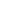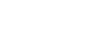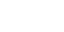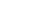# GRE Quantitative Comparison: The Devil is in the Details

Many quantitative comparison questions come with parameters. Parameters are basically a few ground rules that are listed above Column A and Column B.

x is a positive integer

Column A Column B1. The quantity in Column A is greater
2. The quantity in Column B is greater
3. The two quantities are equal
4. The relationship cannot be determined from the information given

In this question x is a positive integer is a parameter. The parameter is essential information. That is you must take into account if you want to get the correct answer.

The answer to the question above is (D). If x = 1, then the columns are equal. For all other values (B) is bigger.

To see how a simple tweak in a parameter can change a question have a look at a similar problem.

x is an integer greater than 1

Column A Column B1. The quantity in Column A is greater
2. The quantity in Column B is greater
3. The two quantities are equal
4. The relationship cannot be determined from the information given

Let’s now look at two questions that are very similar save for a word or two. Both questions are much more difficult so you may want to grab some scratch paper.

1. Isosceles right triangle ABC and Square EFGH have the same area.

Column A Column B
Perimeter of ABC Perimeter of EFGH

1. The quantity in Column A is greater
2. The quantity in Column B is greater
3. The two quantities are equal
4. The relationship cannot be determined from the information given

2. Quadrilateral EFGH and right triangle ABC have the same area.

Column A Column B
Perimeter of ABC Perimeter of EFGH

1. The quantity in Column A is greater
2. The quantity in Column B is greater
3. The two quantities are equal
4. The relationship cannot be determined from the information given

## Solution for #1:

In this question, we have an isosceles right triangle, aka a 45-45-90 triangle. This is fixed triangle—meaning you can’t endlessly manipulate it. Two sides are equal and it is a right triangle, so the sides must be in the ratio of x: x: x√2.

But let’s back up a moment. A square has the same area as our 45-45-90 triangle. At this point, I like to use a convenient number (but if you are predisposed to using ‘x’ then go ahead and do so). Let’s say the area of the square is 8. That means each side of the triangle is 4 (Area of triangle: (4)(4)/2 = 8).

The three sides of the triangle added together will be 4 + 4 + 4√2 = 8 + 4√2.

If the square has an area of 8, each side is equal to √8 = 2√2. The perimeter of the square is 4 x 2√2 = 8√2.

Now we can compare these two numbers:

Column A Column BBe very careful here! First off do not add 8 + 4√2. You cannot add an integer to a number expressed as a radical. One solution:

√2 = 1.4 (approximately)

4 x 1.4 = 5.6

5.6 + 8 = 13.6

8√2 = 8 x 1.4 = 11.2

Column A Column BTherefore (A).

Another solution:

8√2 = 4√2 + 4√2

4√2 + 8 vs. 4√2 + 4√2 (Subtract 4√2 from both sides)

8 vs. 4√2

(4)(2) vs (4)(√2) so (A).

## Solution for #2

A slight (but devilish) change in the details gives us question #2. Note that the square has changed to a quadrilateral and the triangle is now simply a right triangle. Does this change anything?

Imagine that quadrilateral is a square. Imagine that the triangle is an isosceles. We have the first question. How does this help us? Well, in the first question (A) was bigger. If we find an instance in which (A) is not bigger, then the answer is (D).

Imagine the quadrilateral is very skinny, let’s say length is 9 and width is 1. The area would only be 9, but the perimeter would be 20. A triangle with area nine could have sides 3 and 6. Just to equal 20, the triangle would have to have a hypotenuse of 11, which would be impossible: longest possible side of triangle with legs 3 and 6 is < 9.

And like that we’ve found a case where (B) is larger. So the answer is (D).

## Takeaway

On Quantitative Comparison a simple word can change an entire answer.

## Author

•Chris Lele is the Principal Curriculum Manager (and vocabulary wizard) at Magoosh. Chris graduated from UCLA with a BA in Psychology and has 20 years of experience in the test prep industry. He's been quoted as a subject expert in many publications, including US News, GMAC, and Business Because. In his time at Magoosh, Chris has taught countless students how to tackle the GRE, GMAT, SAT, ACT, MCAT (CARS), and LSAT exams with confidence. Some of his students have even gone on to get near-perfect scores. You can find Chris on YouTube, LinkedIn, Twitter and Facebook!